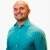# PC Unit 3 Find the missing side of a triangle using the pythagorean theoremThis lesson will look at an example of how to find the missing side of a triangle using the pythagorean theorem.
0 Views